Top 10 Origin Countries of Accepted Refugees

# Number of Refugees accepted by the US (2002 - 2018)

## Packages and Folders

# Install these packages if you don't have theme yet
# devtools::install_github("favstats/tidytemplate")
# install.packages("pacman")

# Creates folders
# tidytemplate::data_dir()
# tidytemplate::images_dir()


refugee_dat <- read_excel("data/refugee_dat.xls") %>%
drop_na(X__1) %>%
rename(cntry = X__1) %>%
select(-Religion, -X__2, -X__3, - Total) %>%
filter(!(str_detect(cntry, "Total|Data"))) %>%
gather(year, n, -cntry) %>%
mutate(year = str_replace(year, "CY ", "") %>% as.numeric)

refugee_dat %>% group_by(cntry) %>% tally() %>% arrange(desc(nn)) %>% .[1:10,] %>% .$cntry -> top10  ## Using n as weighting variable  ## Static year_dat <- tibble(year = c(2005, 2009, 2013, 2017), label = c("Bush II", "Obama I", "Obama II", "Trump I")) refugee_total <- refugee_dat %>% group_by(year) %>% tally() %>% ggplot(aes(year, nn)) + geom_vline(data = year_dat, aes(xintercept = year), alpha = 0.15) + geom_label(data = year_dat, aes(x = year, y = 100000, label = label), angle = 0, color = "black") + geom_line(linetype = "dashed") + geom_point() + theme_minimal() + scale_color_manual("Country", values = qualitative) + theme(plot.title = element_text(size = 13, face = "bold"), plot.subtitle = element_text(size = 11, face = "bold"), plot.caption = element_text(size = 10), legend.position = "bottom") + scale_x_continuous(breaks = 2002:2018, labels = 2002:2018) + labs(x = "", y = "Number of Refugees\n", title = "Accepted Refugees in the United States of America by Year (2002 - 2018)", subtitle = "Total accepted Refugees in Timerange: 943.338\n", caption = "Data: Department of State, Office of Admissions - Refugee Processing Center\n@FabioFavusMaxim; favstats.eu")  ## Using n as weighting variable  refugee_total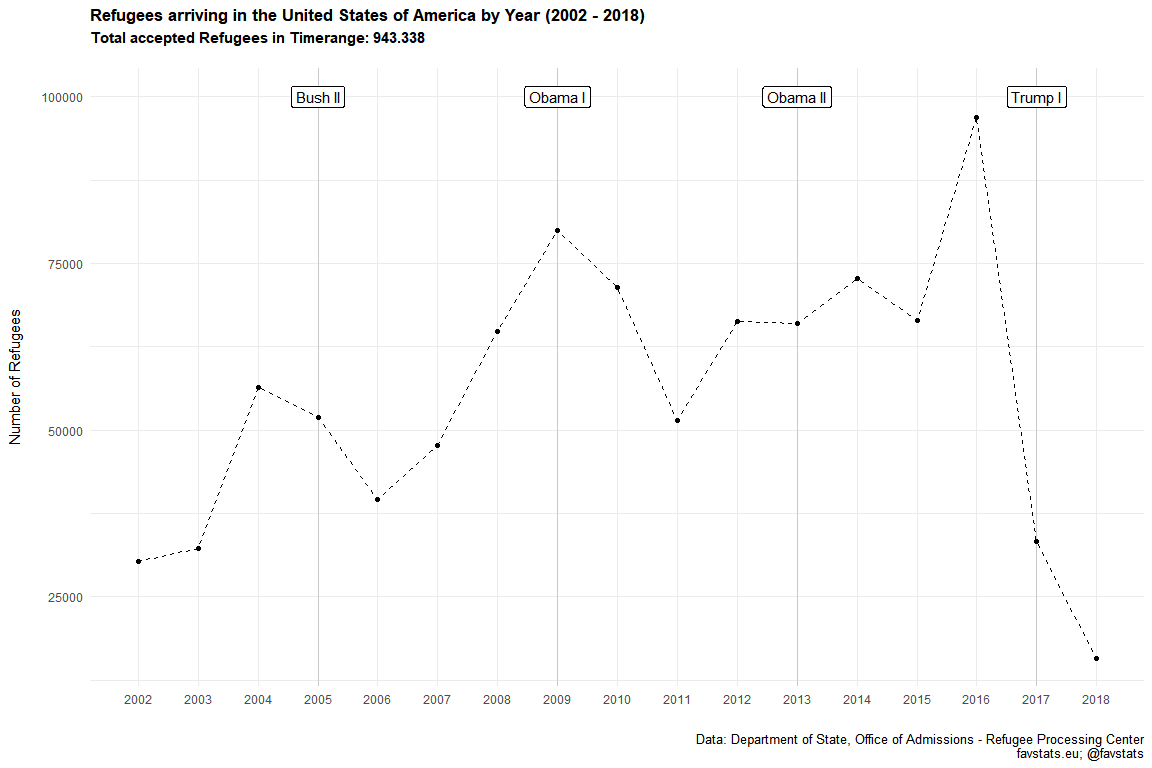tidytemplate::ggsave_it(refugee_total, width = 10, height = 6)  ## Colored qualitative <- c('#a6cee3','#1f78b4','#b2df8a','#33a02c','#fb9a99','#e31a1c','#fdbf6f','#ff7f00','#cab2d6','#6a3d9a') gg_refugee_static_data <- refugee_dat %>% filter(cntry %in% top10) label_dat <- gg_refugee_static_data %>% group_by(cntry) %>% summarize(n = max(n)) %>% select(-cntry) %>% inner_join(gg_refugee_static_data)  ## Joining, by = "n"  gg_refugee_static <- gg_refugee_static_data %>% ggplot(aes(year, n)) + geom_vline(data = year_dat, aes(xintercept = year), alpha = 0.15) + geom_label(data = year_dat, aes(x = year, y = 22000, label = label), angle = 0, color = "black") + geom_line(aes(linetype = cntry, color = cntry)) + theme_minimal() + ggrepel::geom_label_repel(data = label_dat, aes(label = cntry, color = cntry), show.legend = F) + geom_point(data = label_dat, aes(color = cntry)) + scale_color_manual("Country", values = qualitative) + scale_linetype("Country") + theme(plot.title = element_text(size = 13, face = "bold"), plot.subtitle = element_text(size = 11, face = "bold"), plot.caption = element_text(size = 10), legend.key.width = unit(3, "line"), legend.position = "bottom") + scale_x_continuous(breaks = 2002:2018, labels = 2002:2018) + labs(x = "", y = "Number of Refugees\n", title = "Accepted Refugees in the United States of America by Year (2002 - 2018)", subtitle = "Top 10 Origin Countries\n", caption = "Data: Department of State, Office of Admissions - Refugee Processing Center\n@FabioFavusMaxim; favstats.eu") # geom_rect(aes(xmin = 2002, xmax = 2005, ymin = 22000, ymax = 22000), # color = "black", # alpha = 0.8, # inherit.aes = FALSE) gg_refugee_static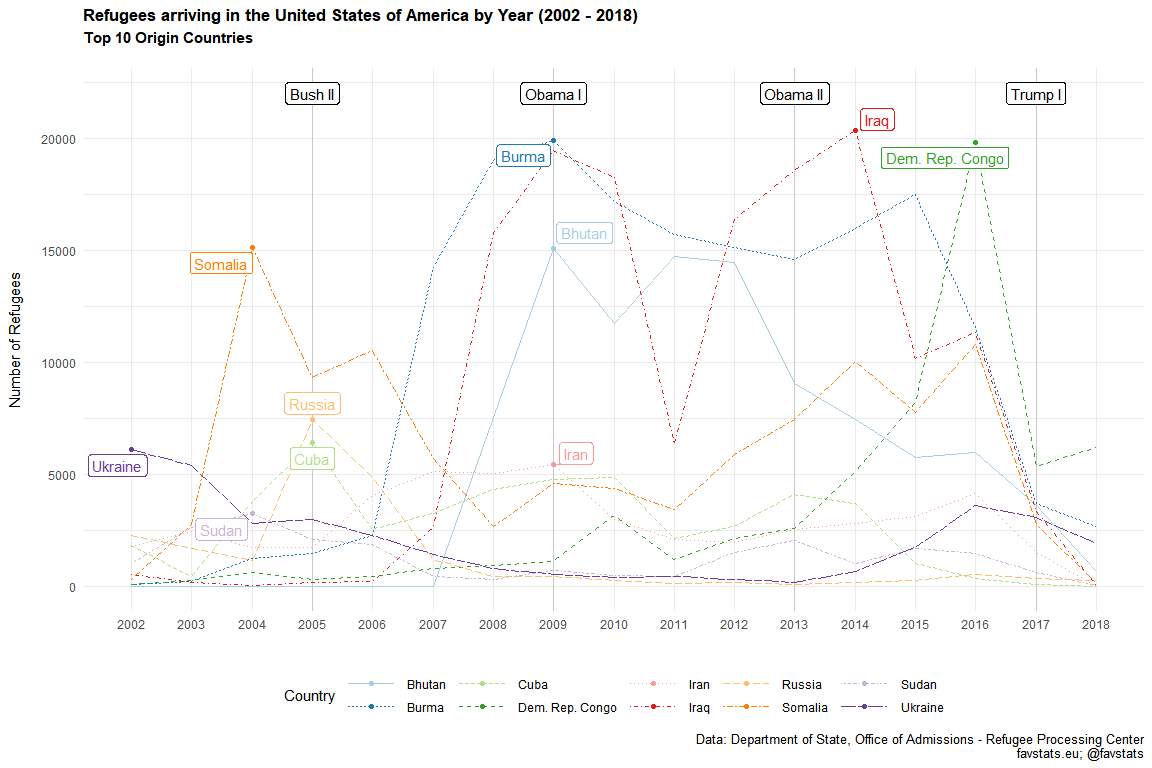tidytemplate::ggsave_it(gg_refugee_static, width = 12, height = 8)  ## Animated library(gganimate) gg_refugee <- refugee_dat %>% filter(cntry %in% top10) %>% ggplot(aes(year, n, color = cntry)) + geom_vline(data = year_dat, aes(xintercept = year), alpha = 0.15) + geom_label(data = year_dat, aes(x = year, y = 22000, label = label), angle = 0, color = "black") + geom_line() + geom_segment(aes(xend = 2018, yend = n), alpha = 0.5) + geom_point() + geom_text(aes(x = 2019, label = cntry)) + theme_minimal() + scale_color_manual("Country", values = qualitative) + theme(plot.title = element_text(size = 13, face = "bold"), plot.subtitle = element_text(size = 12, face = "bold"), plot.caption = element_text(size = 10), legend.position = "bottom") + scale_x_continuous(breaks = 2002:2018, labels = 2002:2018) + labs(x = "", y = "Number of Refugees\n", title = "Accepted Refugees in the United States of America by Year (2002 - 2018)", subtitle = "Top 10 Origin Countries\n", caption = "Data: Department of State, Office of Admissions - Refugee Processing Center\n@FabioFavusMaxim; favstats.eu") + guides(color = F, text = F) + transition_reveal(cntry, year, keep_last = T) gg_refugee %>% animate( nframes = 500, fps = 15, width = 1000, height = 600, detail = 1 ) anim_save("images/gg_refugee.gif")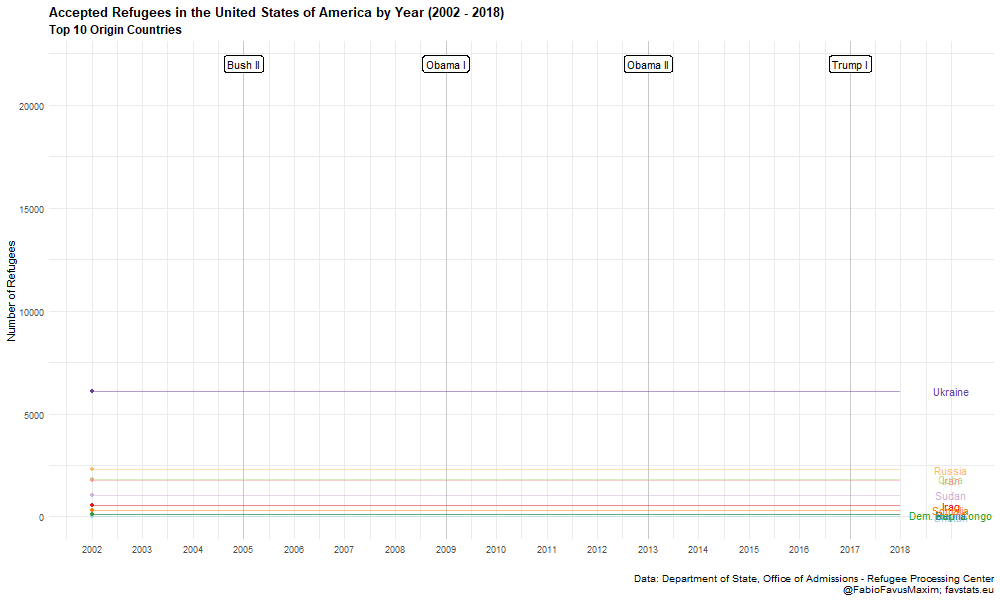## Region ### Static gg_refugee_static_region <- refugee_dat %>% mutate(continent = countrycode::countrycode(cntry, "country.name", "continent")) %>% mutate(region = countrycode::countrycode(cntry, "country.name", "region")) %>% mutate(continent = case_when( continent == "Asia" ~ region, T ~ continent )) %>% mutate(continent = case_when( cntry == "Tibet" ~ "Eastern Asia", cntry == "Yemen (Sanaa)" ~ "Middle East", cntry == "Yugoslavia" ~ "Europe", str_detect(cntry, "Georgia|Armenia|Azerbaijan") ~ "Central Asia", continent == "Western Asia" ~ "Middle East", T ~ continent )) %>% # group_by(continent) %>% tally # filter(continent == "Western Asia") group_by(year, continent) %>% tally()  ## Warning in countrycode::countrycode(cntry, "country.name", "continent"): Some values were not matched unambiguously: Tibet, Yemen (Sanaa), Yugoslavia ## Warning in countrycode::countrycode(cntry, "country.name", "region"): Some values were not matched unambiguously: Tibet, Yemen (Sanaa), Yugoslavia ## Using n as weighting variable  # gg_refugee_static_region %>% filter(continent == "Southern Asia") %>% summarize_all(sum) label_dat <- gg_refugee_static_region %>% group_by(continent) %>% summarize(nn = max(nn)) %>% select(-continent) %>% inner_join(gg_refugee_static_region) %>% filter(!(year == 2018 & continent == "Eastern Asia")) %>% filter(!(year == 2004 & continent == "Eastern Asia")) %>% mutate(nn = ifelse(continent == "Southern Asia", 10385, nn)) %>% mutate(year = ifelse(continent == "Southern Asia", 2015, year))  ## Joining, by = "nn"  gg_static_region <- gg_refugee_static_region %>% ggplot(aes(year, nn)) + geom_vline(data = year_dat, aes(xintercept = year), alpha = 0.15) + geom_label(data = year_dat, aes(x = year, y = 41000, label = label), angle = 0, color = "black") + geom_line(aes(linetype = continent, color = continent)) + theme_minimal() + ggrepel::geom_label_repel(data = label_dat, aes(label = continent, color = continent), show.legend = F, seed = 13092018) + geom_point(data = label_dat, aes(color = continent)) + scale_color_manual("Region", values = qualitative) + scale_linetype("Region") + theme(plot.title = element_text(size = 13, face = "bold"), # plot.subtitle = element_text(size = 11, face = "bold"), plot.caption = element_text(size = 10), legend.key.width = unit(3, "line"), legend.position = "bottom") + scale_x_continuous(breaks = 2002:2018, labels = 2002:2018, minor_breaks = seq(2002, 2018, 1)) + labs(x = "", y = "Number of Refugees\n", title = "Refugees arriving in the United States of America by Year (2002 - 2018)", caption = "Data: Department of State, Office of Admissions - Refugee Processing Center\nfavstats.eu; @favstats") # geom_rect(aes(xmin = 2002, xmax = 2005, ymin = 22000, ymax = 22000), # color = "black", # alpha = 0.8, # inherit.aes = FALSE) gg_static_region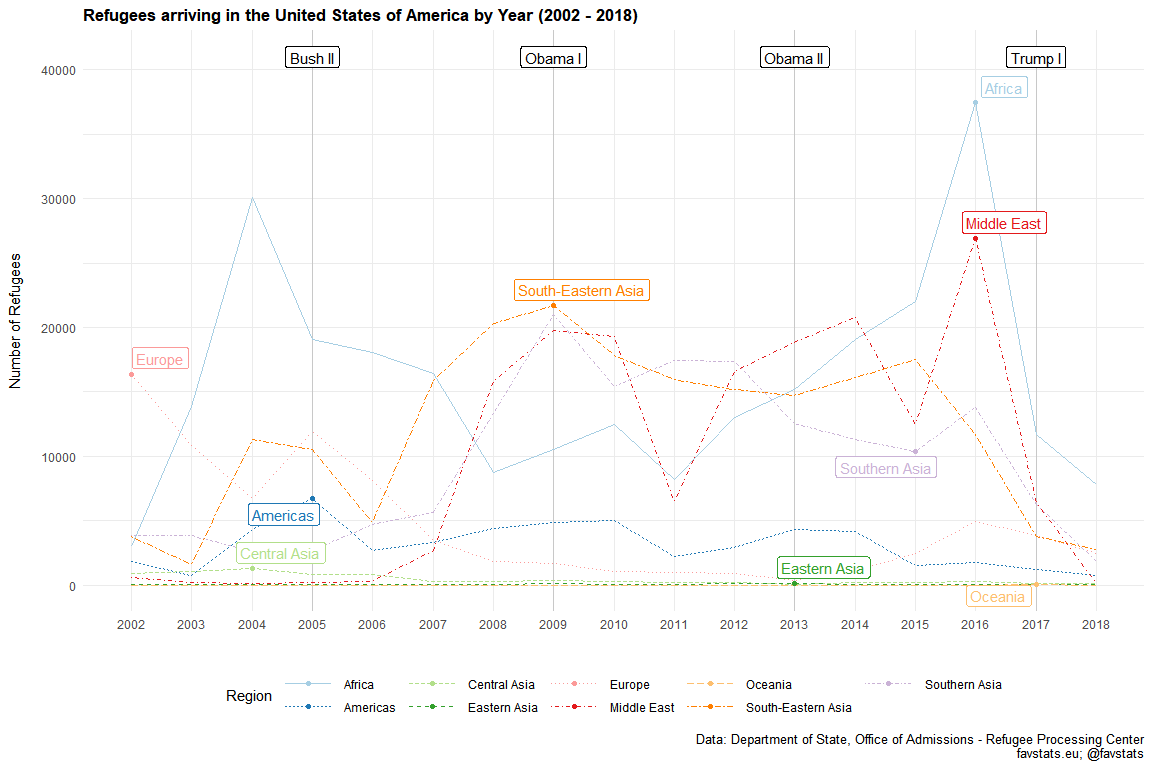tidytemplate::ggsave_it(gg_static_region, width = 12, height = 8)  ### Percent gg_static_region_perc <- gg_refugee_static_region %>% group_by(year) %>% mutate(total = sum(nn)) %>% mutate(perc = tidytemplate::get_percentage(nn, total)) label_dat <- gg_static_region_perc %>% group_by(continent) %>% summarize(perc = max(perc)) %>% select(-continent) %>% inner_join(gg_static_region_perc)  ## Joining, by = "perc"  gg_region_perc <- gg_static_region_perc %>% ggplot(aes(year, perc)) + geom_vline(data = year_dat, aes(xintercept = year), alpha = 0.15) + geom_label(data = year_dat, aes(x = year, y = 60, label = label), angle = 0, color = "black") + geom_line(aes(linetype = continent, color = continent)) + theme_minimal() + ggrepel::geom_label_repel(data = label_dat, aes(label = continent, color = continent), show.legend = F, seed = 13092018) + geom_point(data = label_dat, aes(color = continent)) + scale_color_manual("Region", values = qualitative) + scale_linetype("Region") + theme(plot.title = element_text(size = 13, face = "bold"), # plot.subtitle = element_text(size = 11, face = "bold"), plot.caption = element_text(size = 10), legend.key.width = unit(3, "line"), legend.position = "bottom") + scale_x_continuous(breaks = 2002:2018, labels = 2002:2018, minor_breaks = seq(2002, 2018, 1)) + labs(x = "", y = "Percentage of Refugees\n", title = "Refugees arriving in the United States of America by Year (2002 - 2018)\n", caption = "Data: Department of State, Office of Admissions - Refugee Processing Center\nfavstats.eu; @favstats") gg_region_perc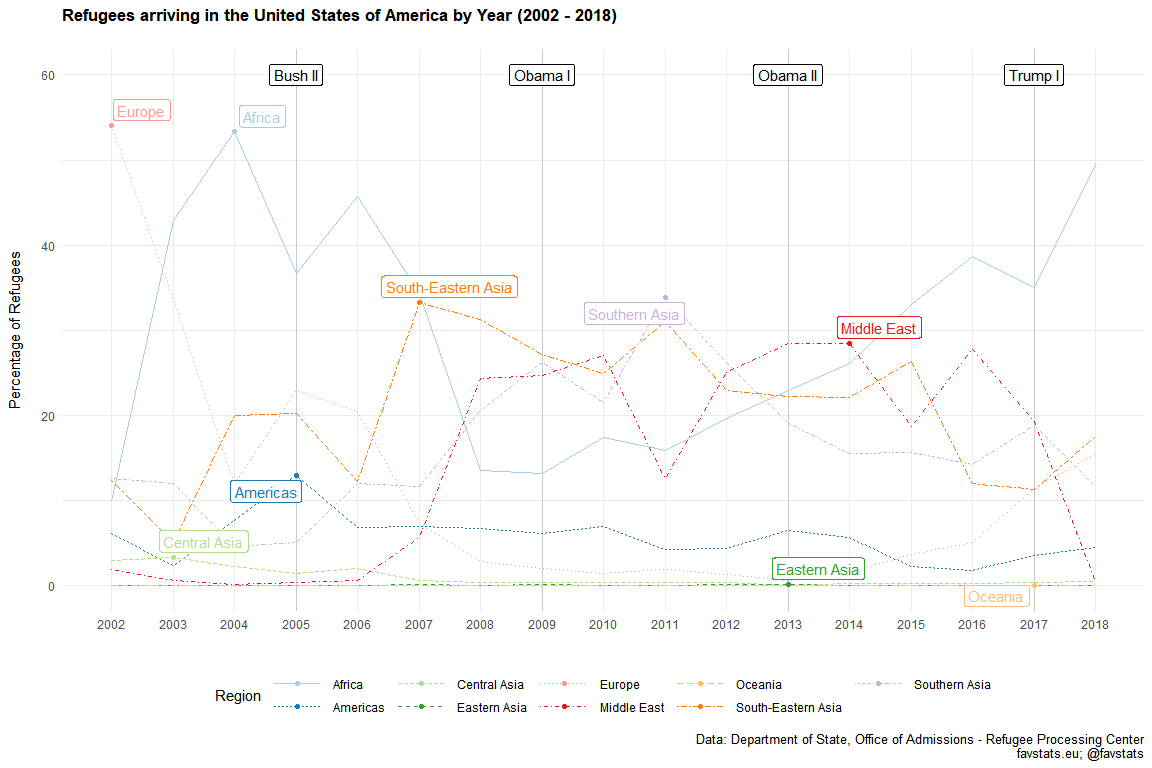tidytemplate::ggsave_it(gg_region_perc, width = 12, height = 8)  ### Animation library(gganimate) gg_anim_region <- gg_refugee_static_region %>% mutate(continent = ifelse(continent == "South-Eastern Asia", "S.E. Asia", continent)) %>% ggplot(aes(year, nn, color = continent)) + geom_vline(data = year_dat, aes(xintercept = year), alpha = 0.15) + geom_label(data = year_dat, aes(x = year, y = 41000, label = label), angle = 0, color = "black") + geom_line() + geom_segment(aes(xend = 2018, yend = nn), alpha = 0.5) + geom_point() + geom_text(aes(x = 2019, label = continent)) + theme_minimal() + scale_color_manual("Country", values = qualitative) + theme(plot.title = element_text(size = 13, face = "bold"), # plot.subtitle = element_text(size = 12, face = "bold"), plot.caption = element_text(size = 10), legend.position = "bottom") + scale_x_continuous(breaks = 2002:2018, labels = 2002:2018, minor_breaks = seq(2002, 2018, 1)) + labs(x = "", y = "Number of Refugees\n", title = "Refugees arriving in the United States of America by Year (2002 - 2018)\n", caption = "Data: Department of State, Office of Admissions - Refugee Processing Center\nfavstats.eu; @favstats") + guides(color = F, text = F) + transition_reveal(continent, year, keep_last = T) gg_anim_region %>% animate( nframes = 500, fps = 15, width = 1000, height = 600, detail = 1 ) anim_save("images/gg_anim_region.gif")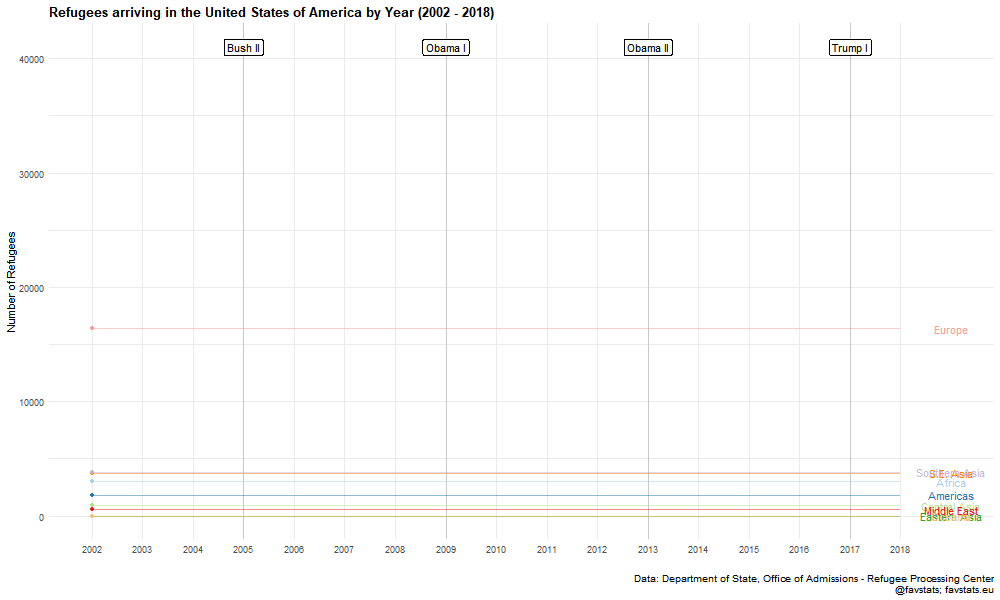## Religion Data relig_refugee <- read_excel("data/refugee_dat.xls") %>% drop_na(Religion) %>% rename(religion = Religion) %>% select(-X__1, -X__2, -X__3, - Total) %>% # filter(!(str_detect(cntry, "Total|Data"))) %>% gather(year, n, -religion) %>% mutate(year = str_replace(year, "CY ", "") %>% as.numeric) %>% # group_by(religion, year) %>% # mutate(n = sum(n)) %>% mutate(religion_cat = case_when( str_detect(religion, "Moslem|Ahmadiyya") ~ "Muslim", str_detect(religion, "Christ|Baptist|Chald|Coptic|Greek|Jehovah|Lutheran|Mennonite|Orthodox|Pentecostalist|Protestant|Uniate|Adventist|Cath|Meth|Old Believer") ~ "Christian", str_detect(religion, "Atheist|No Religion") ~ "Atheist/No Religion", religion == "Hindu" ~ "Hindu", T ~ "Other/Unknown" )) %>% # filter(religion == "Unknown") %>% # .$n %>% sum%>%
group_by(religion_cat, year) %>%
summarize(n = sum(n))


### Static

label_dat <- relig_refugee %>%
filter(year == 2018)

gg_relig <- relig_refugee  %>%
ggplot(aes(year, n)) +
geom_vline(data = year_dat, aes(xintercept = year), alpha = 0.15) +
geom_label(data = year_dat, aes(x = year, y = 48000, label = label),
angle = 0, color = "black") +
geom_line(aes(color = religion_cat), size = .8) +
theme_minimal() +
ggrepel::geom_text_repel(data = label_dat,
aes(label = religion_cat, color = religion_cat),
nudge_x = 2,
show.legend = F,
direction = "y", min.segment.length = 0.7) +
geom_point(data = label_dat, aes(color = religion_cat)) +
ggthemes::scale_color_gdocs("Religion") +
# scale_color_manual("Religion", values = qualitative) +
scale_linetype("Religion") +
theme(plot.title = element_text(size = 13, face = "bold"),
plot.subtitle = element_text(size = 11, face = "bold"),
plot.caption = element_text(size = 10),
legend.key.width = unit(3, "line"),
legend.position = "bottom") +
scale_x_continuous(breaks = 2002:2018, labels = 2002:2018,
limits = c(2002, 2021),
minor_breaks = seq(2002, 2018, 1)) +
labs(x = "", y = "Number of Refugees\n",
title = "Refugees arriving in the United States of America by Year (2002 - 2018)", subtitle = "Aggregated by Religion\n",
caption = "Data: Department of State, Office of Admissions - Refugee Processing Center\nfavstats.eu; @favstats") +
guides(color = F)

gg_relig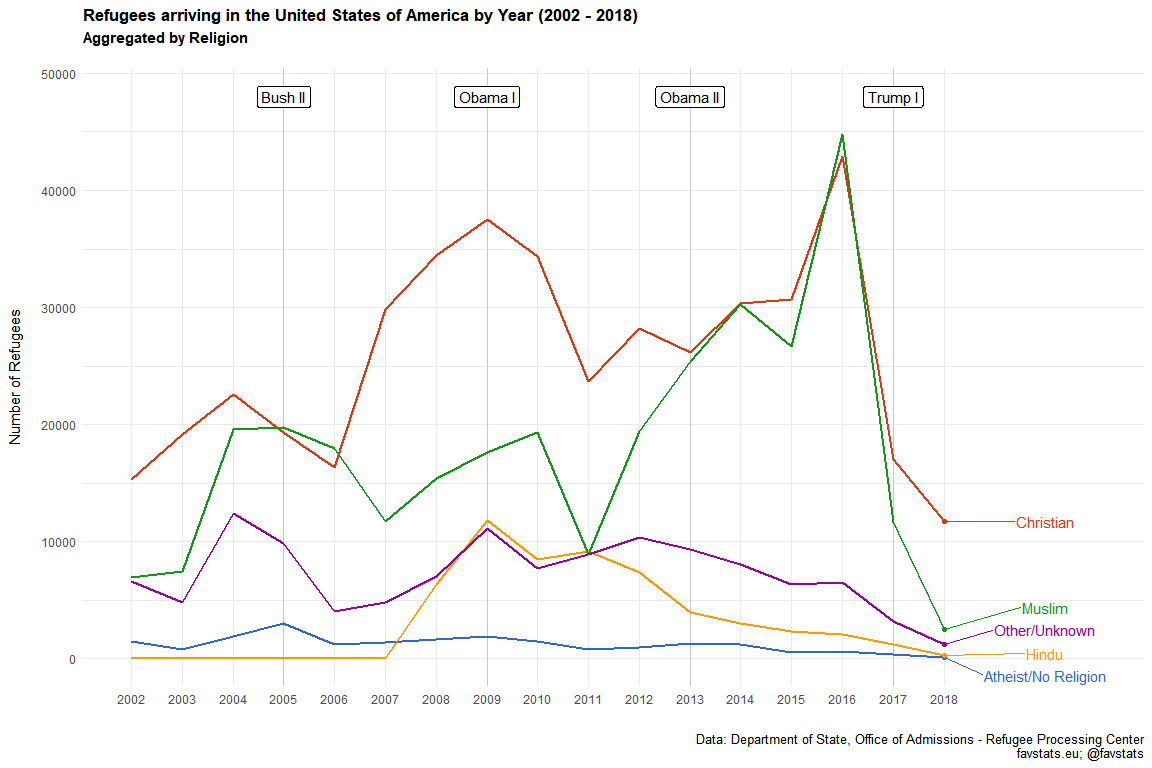tidytemplate::ggsave_it(gg_relig, width = 10, height = 6)


### Animated

library(gganimate)

gg_religion <- relig_refugee %>%
ggplot(aes(year, n, color = religion_cat)) +
geom_vline(data = year_dat, aes(xintercept = year), alpha = 0.15) +
geom_label(data = year_dat, aes(x = year, y = 48000, label = label),
angle = 0, color = "black", size = 6) +
geom_line(size = 1) +
geom_segment(aes(xend = 2018, yend = n), alpha = 0.5) +
geom_point(size = 2) +
geom_text(aes(x = 2019, label = religion_cat),
size = 6, face = "bold", nudge_x = .75) +
theme_minimal() +
ggthemes::scale_color_gdocs("Religion") +
theme(plot.title = element_text(size = 18, face = "bold"),
plot.subtitle = element_text(size = 16, face = "bold"),
plot.caption = element_text(size = 14),
axis.title = element_text(size = 14, face = "bold"),
axis.text = element_text(size = 14),
legend.position = "bottom") +
scale_x_continuous(breaks = 2002:2018,
labels = c("'02", "'03", "'04",
"'05", "'06", "'07",
"'08", "'09", "'10",
"'11", "'12", "'13",
"'14", "'15", "'16",
"'17", "'18"),
limits = c(2002, 2020.5),
minor_breaks = seq(2002, 2018, 1)) +
labs(x = "", y = "Number of Refugees\n\n",
title = "Refugees arriving in the United States of America by Year (2002 - 2018)", subtitle = "Aggregated by Religion\n\n",
caption = "Data: Department of State, Office of Admissions - Refugee Processing Center\nfavstats.eu; @favstats")  +
guides(color = F, text = F) +
transition_reveal(religion_cat, year, keep_last = T)

gg_religion %>% animate(
nframes = 500, fps = 15, width = 1000, height = 600, detail = 1
)

anim_save("images/gg_religion.gif")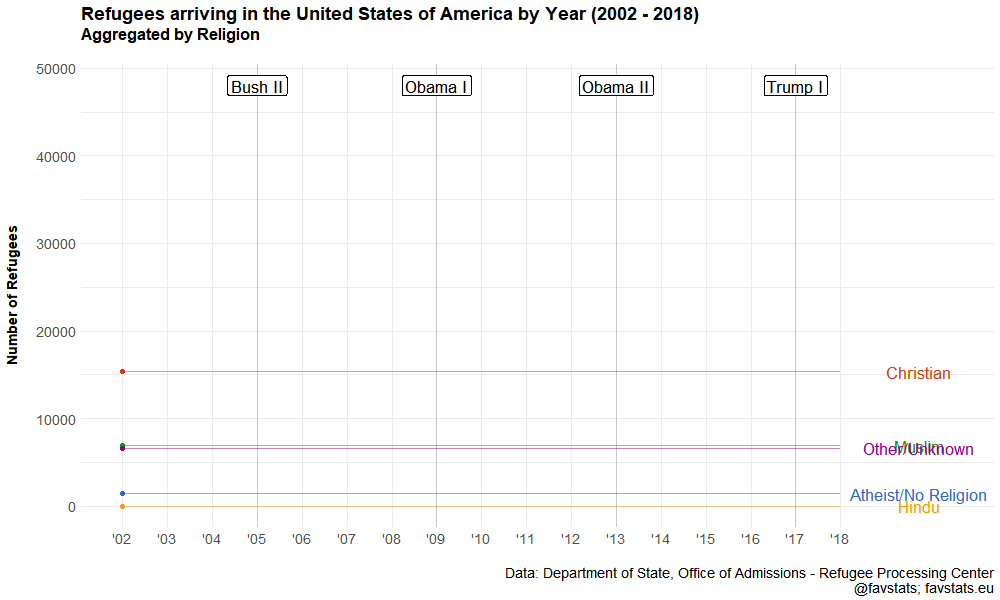## Maps

load("data/world_map.Rdata")

refugee_map_total <- refugee_dat %>%
mutate(id = countrycode::countrycode(cntry, "country.name", "country.name")) %>%
mutate(id = ifelse(cntry == "Tibet", yes = "China", no = id)) %>%
group_by(id) %>%
summarize(n = sum(n, na.rm = T)) %>%
full_join(world_map) %>%
mutate(n = cut(n,
breaks = c(1, 100, 10000, 50000, 100000, 175000),
labels = c("< 100", "100 - 10.000",
"10.001 - 50.000", "50.001 - 100.000",
"100.001 - 175.000")))


### Total Map

refugee_map_total %>%
ggplot() +
geom_map(map = world_map,
aes(x = long, y = lat, group = group, map_id = id),
color = "#7f7f7f", fill = "gray80", size = 0.15) +
geom_map(data = refugee_map_total,
map = world_map,
aes(map_id  = id,
fill = n), size = 0.01) +
theme_void() +
#  scale_fill_gradient(low = "red", high = "blue") +
coord_equal() +
viridis::scale_fill_viridis("Number of Refugees",
direction = -1,
option = "D",
discrete = T,
# begin = .2,
# end = .8,
na.value = "grey",
#       limits = c(0, 1),
# breaks = c(0, .20, .40, .60, .8, 1),
labels = c("< 100", "100 - 10.000",
"10.000 - 50.000", "50.000 - 100.000",
"100.000 - 175.000", "No Refugees")) +
# facet_wrap(~year, ncol = 6) +
theme(
plot.title = element_text(size = 18, face = "bold", hjust = 0.5),
plot.caption = element_text(size = 14),
legend.justification = c(1, 0),
legend.position = c(0.2, 0.25),
legend.title = element_text(size = 10),
#axis.ticks.length = unit(3, "cm"),
legend.direction = "vertical") +
# guides(fill = guide_colorbar(barwidth = 0.7, barheight = 15,
#               title.position = "bottom", title.hjust = 0.5,
#               label.theme = element_text(colour = "black", size = 6, angle = 0))) +
labs(x = "", y = "",
title = "Refugees arriving in the United States of America by Nationality (2002 - 2018)",
caption = "Data: Department of State, Office of Admissions - Refugee Processing Center   \nfavstats.eu; @favstats   ")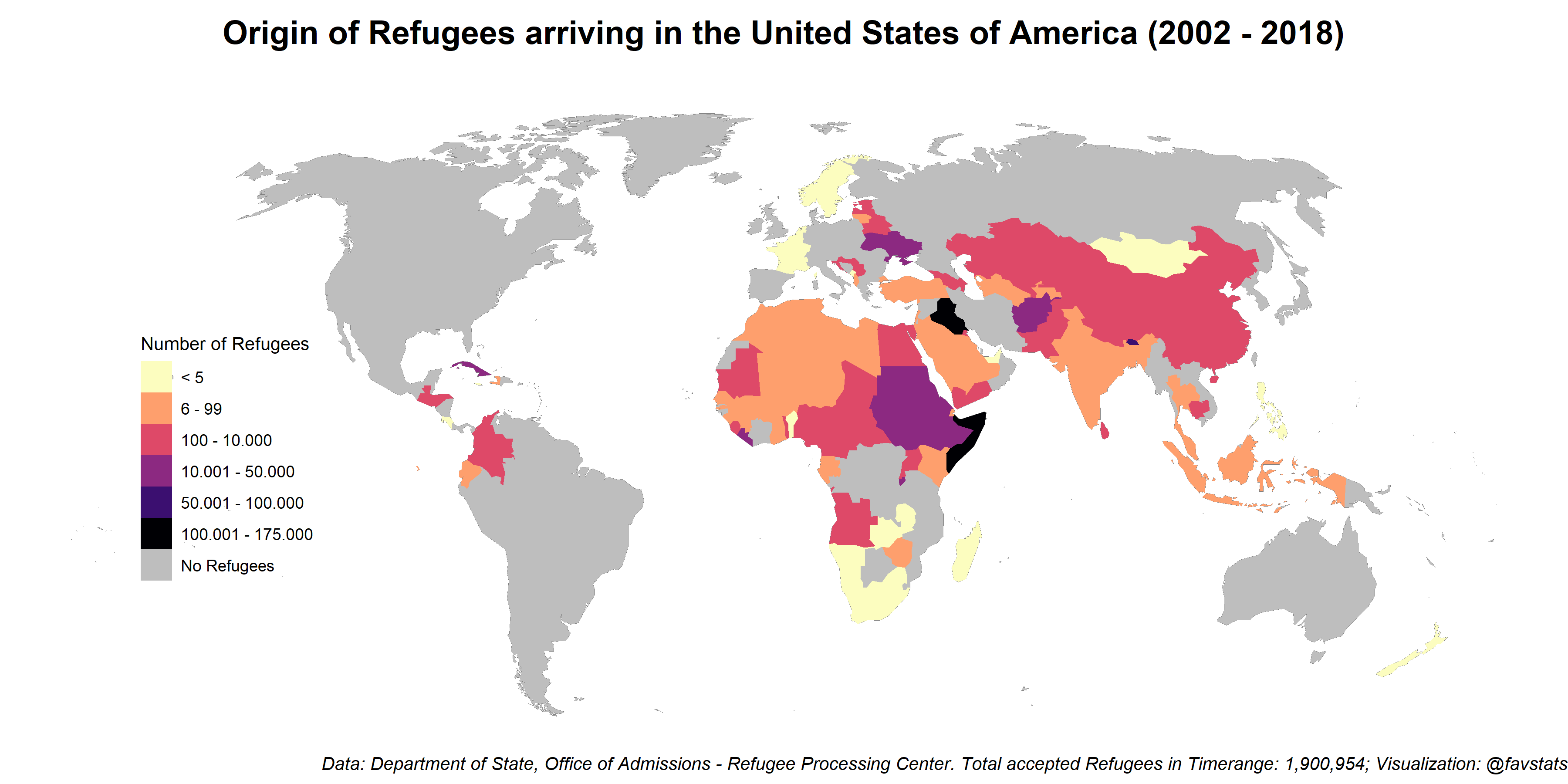ggsave(filename = "images/refugee_total_map.png", height = 6, width = 12)



## Extended Data (1975 - 2018)

refuge_admissions75 <- 'https://static1.squarespace.com/static/580e4274e58c624696efadc6/t/5b8ff632aa4a999f85f99e8d/1536161330411/Graph+Refugee+Admissions+since+1975%289.5.18%29.pdf'

# Extract the table

first_pages <- do.call(rbind, out[-length(out)]) %>% as_tibble()

correct_names <- first_pages[2,] %>% as.character()

final1 <- first_pages %>%
set_names(correct_names) %>%
.[-c(1:2),] %>%
.[-c(length(.)),]

last_page <- cbind(
out[][,1],
out[][,2],
out[][,3],
out[][,5],
out[][,6],
out[][,7],
out[][,8],
out[][,9],
out[][,10],
out[][,11]
) %>% as_tibble()

correct_lastpage <- last_page[2,] %>% as.character()

final2 <- last_page %>%
set_names(correct_lastpage) %>%
.[-c(1:2),]

select(-Total) %>%
mutate_all(parse_number) %>%
na.omit() %>%
gather(region, n, -Fiscal\rYear) %>%
janitor::clean_names()


### Plot It

year_lab <- paste0("'", stringi::stri_sub(1975:2018, -2 , -1))

year_dat <- tibble(fiscal_year = c(seq(1976, 2016, 4)),
label = c("Carter I", "Reagan I", "Reagan II",
"H.W. Bush I", "Clinton I", "Clinton II", "Bush I",
"Bush II", "Obama I", "Obama II", "Trump I"))

filter(fiscal_year == 2018) %>%
summarize(n = sum(n)) %>%
.$n n_refugee_2002 <- admissions75 %>% filter(fiscal_year == 2002) %>% summarize(n = sum(n)) %>% .$n

filter(fiscal_year == 1992) %>%
summarize(n = sum(n)) %>%
.$n n_refugee_1980 <- admissions75 %>% filter(fiscal_year == 1980) %>% summarize(n = sum(n)) %>% .$n

filter(fiscal_year == 1975) %>%
summarize(n = sum(n)) %>%
.$n admissions75 %>% summarize(n = sum(n)) %>% .$n

mutate(region = case_when(
region == "Former\rSoviet\rUnion" ~ "(Former) Soviet Union",
region == "Latin America\rCaribbean" ~ "Latin America/Caribbean",
region == "Near East\rSouth Asia" ~ "Near East/South Asia",
region == "PSI" ~ "Private Sector Initiative",
T ~ region
)) %>%
ggplot(aes(fiscal_year, n))  +
geom_vline(data = year_dat, aes(xintercept = fiscal_year), alpha = 0.35) +
geom_label(data = year_dat, aes(x = fiscal_year, y = 220000, label = label),
angle = 0, color = "black") +
geom_area(aes(fill = region), alpha = 0.9) +
geom_hline(yintercept = n_refugee_2018,
linetype = "dashed", color = "black", alpha = 0.85) +
annotate("label", x = 1978, y = 115000,
fill = "lightgrey", alpha = 0.85, label.size = NA,
label = "End of\n Vietnam War") +
annotate("label", x = 1984, y = 185000,
fill = "lightgrey", alpha = 0.85, label.size = NA,
label = "Refugee Act of 1980") +
annotate("label", x = 1997, y = 150000,
fill = "lightgrey", alpha = 0.85, label.size = NA,
label = "Fall of Soviet Union") +
annotate("label", x = 2000, y = 105000,
fill = "lightgrey", alpha = 0.85, label.size = NA,
label = "Drop after 9/11") +
annotate("label", x = 2015, y = 110000,
fill = "lightgrey", alpha = 0.85, label.size = NA,
label = "Number of Refugees in 2018\n lowest since 1977") +
theme_minimal() +
scale_y_continuous(labels = scales::comma) +
scale_fill_manual("Region", values = qualitative) +
geom_curve(aes(x = 1977, y = 125000, xend = 1975, yend = n_refugee_1975),
arrow = arrow(length = unit(0.03, "npc")), curvature = 0.2) +
geom_curve(aes(x = 1982, y = 190000, xend = 1980, yend = n_refugee_1980),
arrow = arrow(length = unit(0.03, "npc")), curvature = 0.2) +
geom_curve(aes(x = 1994, y = 150000, xend = 1992, yend = n_refugee_1992),
arrow = arrow(length = unit(0.03, "npc")), curvature = 0.2) +
geom_curve(aes(x = 2000, y = 100000, xend = 2002, yend = n_refugee_2002),
arrow = arrow(length = unit(0.03, "npc")), curvature = -0.3) +
geom_curve(aes(x = 2016, y = 100000, xend = 2018, yend = n_refugee_2018),
arrow = arrow(length = unit(0.03, "npc")), curvature = -0.2) +
theme(plot.title = element_text(size = 13, face = "bold"),
# plot.subtitle = element_text(size = 11, face = "bold"),
plot.caption = element_text(size = 10),
legend.key.width = unit(3, "line"),
legend.position = "bottom") +
scale_x_continuous(breaks = 1975:2018, labels = year_lab,
minor_breaks = seq(1975, 2018, 1)) +
labs(x = "", y = "Number of Refugees\n",
title = "Refugees arriving in the United States of America by Year (1975 - 2018)\n",
caption = "Data: Department of State, Office of Admissions - Refugee Processing Center\nTotal Number of Accepted Refugees since 1975: 3.340.709\nfavstats.eu; @favstats")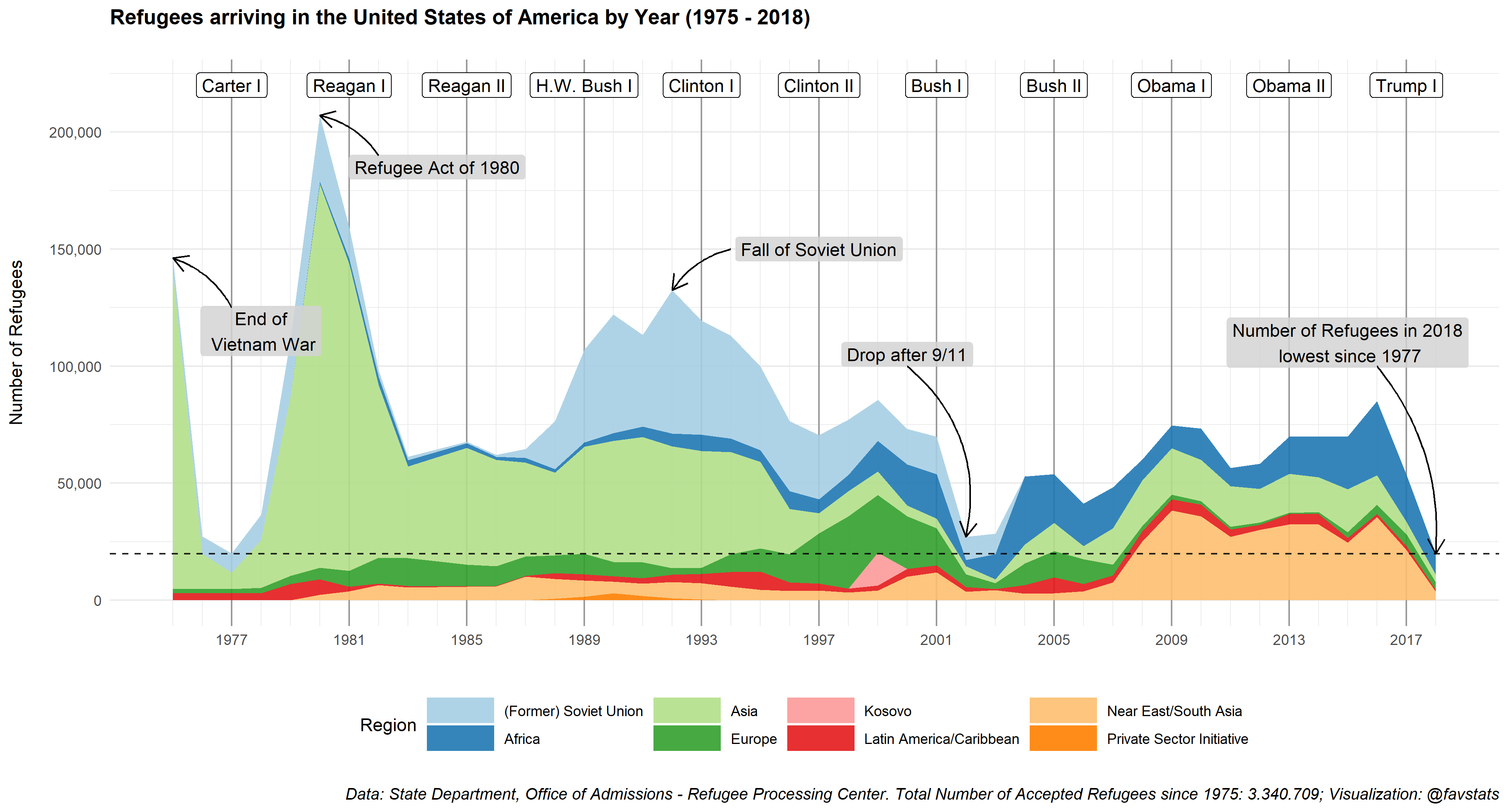ggsave(filename = "images/refugee75.png", height = 7, width = 13)